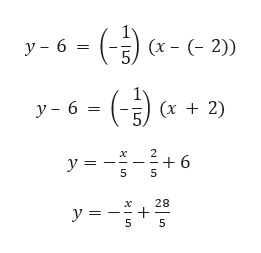# Write an equation in​ slope-intercept form and in standard form for the line passing through (-2,6) and parallel to x+5y=7.

Question

Write an equation in​ slope-intercept form and in standard form for the line passing through (-2,6) and parallel to x+5y=7.

check_circleExpert Solution
Step 1

Given,

line passing through (-2,6) and parallel to x + 5y = 7

Since, slope of the parallel lines are equal.

x + 5y = 7

5y = – x + 7

y = (–1/5) x + (7/5) which is slope-intercept form.

Slope = –1/5

So, slope of the required line is –1/5.

Step 2

Therefore, equation of the line passin...help_outlineImage Transcriptionclose(-Э) (« - (х - (- 2)) у- 6 — (Э (х + 2) у- 6 3 5) х У — -6 5 у3 5 28 х у3 5 5 fullscreen

### Want to see the full answer?

See Solution

#### Want to see this answer and more?

Solutions are written by subject experts who are available 24/7. Questions are typically answered within 1 hour*

See Solution
*Response times may vary by subject and question
Tagged in

### Equations and In-equations